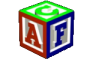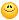Density confusion Author Message
3DRaddictMemberPost: #1 Density confusion I was a little confused by the different "Density" terms (circled below) which appear when using the Object Editor and Physics Material Editor:I checked in the API for references to PhysBody density and found: PHP Code: `Flt            density ; // mass density multiplier, 0..Inf, default=1 ` PHP Code: `Flt  finalDensity()C; // get density of PhysBody including material -> "density * material.density" ` From this I have concluded that the "Density" referred to in the Object Editor is the "mass density multiplier" (no units) and the "Density" referred to in Physics Material Editor is the actual "material.density" (Kg/m3) To ascertain the correctness of my assumption I took the Esenthel capsule as an example to calculate its theoretical mass and then compare with its mass as determined by Esenthel function (actor.mass()) The formula for determining Mass(Kg) is: Density * Volume (where Density has units Kg/m3 and Volume has units m3) The default size of the capsule is 0.5m radius and 1m height for the cylindrical part. The formula for the volume of a capsule is: ( Volume of the two hemispherical ends) + (Volume of cylindrical section) = (Volume of sphere) + (Volume of cylinder) = (1.33 * PI * radius * radius * radius) + (PI * radius * radius * height) Therefore, for our default capsule: Volume = (1.33 * PI * 0.5 * 0.5 * 0.5) + (PI * 0.5 * 0.5 * 1) = 0.522 + 0.786 = 1.308 m3 I've taken the capsule to be made from aluminium material which has a Density of 2700 Kg/m3 Therefore, the Mass of the Capsule is (theoretically) 1.308 * 2700 = 3530 Kg To check this with the Esenthel derived mass (using 1.0 as the "mass density multiplier" and 2700.0 as "material.density") I obtained a result of 3534 Kg, using the function actor.mass() in code. This checks out pretty well with the theoretical value as calculated above. My conclusions from this little exercise are: 1) Esenthel should change the "Density" text in the Object Editor to "Density Multiplier" 2) Users should set the real world value of the density of the Physics Material using the Physics Material Editor using Kg/m3 units 3) Users should keep the default setting of 1.0 for the "Density Multiplier" in the Object Editor (only change this value if you want to fine tune your Material Density) 4) By keeping to the above procedure, your masses will be correct for your basic physbody shapes, which is so important when involving physics in your application. To conclude, here are some real world densities (in Kg/m3) for a few of the Physics Materials that I use: Aluminium : 2700 Brass : 8725 Iron : 7800 Leather : 860 Rubber : 1200 Wood(heavy) : 990 Wood(light) : 500 Water(default) : 1000
05-15-2015 01:31 PM
Pixel PerfectMemberPost: #2 RE: Density confusion As usual, really useful information. Thanks again 3DRaddict05-15-2015 09:38 PM
 « Next Oldest | Next Newest »